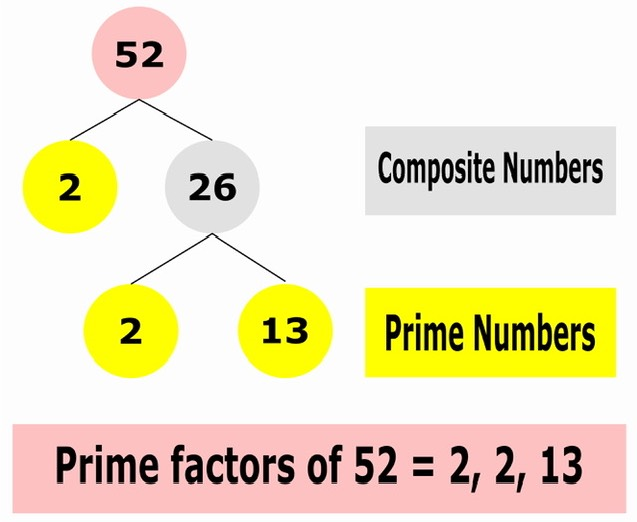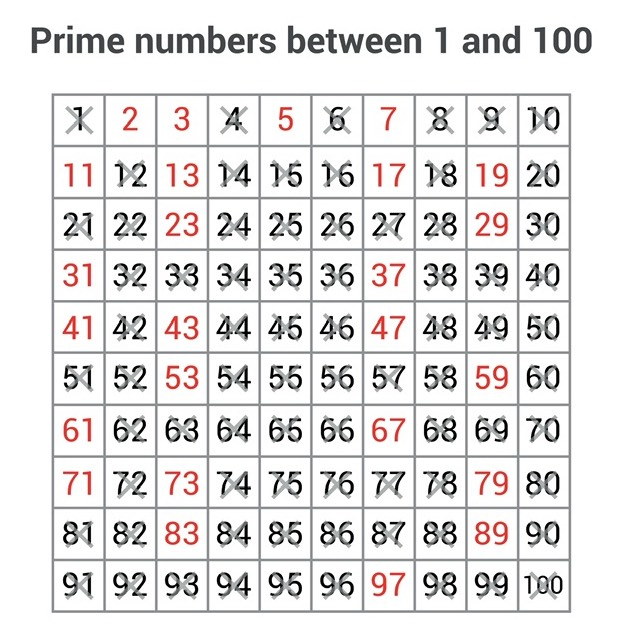×## Introduction

Based on their factor, the prime and composite numbers are distinguished. The composite numbers have more than two factors, whereas the prime numbers have just two. A factor is an integer if it splits a number, leaving zero as the remainder. With cases that have been answered, we will learn about the number system, prime numbers, composite numbers, factors, and the basic idea of arithmetic in this article.

## Number System

A mathematical word with a specific meaning called a number is used to tally or measure things. Mathematics uses a variety of numbers, including natural, fractions, rational, and irregular numbers. The definition of the number system, on the other hand, is the depiction of numbers in different ways that computers can understand. The number system is another name for the numeral system. In mathematics, there are four different kinds of number systems, including

## Prime Numbers

The positive numbers (greater than 1) with only two factors, such as 1 and the number itself, are known as prime numbers. In other words, the prime numbers can be stated only as a whole because they can be divided into equal portions. seven is a significant number, for instance. You can calculate it as

1 х 7 = 7

7 х 1 = 7

Hence it has only two factors, such as 1 and 7.Moreover, prime numbers are an important part of number theory. The prime numbers are the core of cryptography and coding. It is difficult to encrypt the data in a computer without a prime number. Moreover, prime numbers have several properties, which are described below.

• The prime numbers are divisible by only two numbers (i.e., 1 and the number itself).

• A prime number is always greater than 1.

• There exists only one even prime number, such as 2.

• Each and every number can be factorized in terms of prime numbers.

Any two prime numbers are always coprime to each other.

## Composite Numbers

Positive numbers with more than two variables are considered compound numbers. In other terms, a number is referred to as a composite number if it is not a prime number. For example, 6  is a composite number.

Factor of 6 = 1, 2, 3 and 4.

Some more characteristics of the composite numbers are:

• The prime or composite numbers can divide the composite numbers.

• Each composite number is formed from two or more prime integers.

• For example, 8 = 23

• The number system uses two different kinds of composite  numbers.

• Even composite numbers: For example, 4, 6, 8, 10, etc.

• Odd composite numbers: For example, 9, 15, 21, etc.

coprime numbers

The two numbers that have only one factor in common, which is 1, are known as coprime numbers, mutually prime numbers, or relatively prime numbers. Let's use an illustration to better grasp the idea.

For example: 16  and 15.

The factors of 16 =1, 2, 4, 8 and 16

The factors of 15 =  1, 3 and 5

We can see that the common component for the two integers is 1.  Hence, 16 and 15 are therefore coprime numbers.

## Factors

When a factor splits a number, leaving zero as the remainder, it is said to be an integer. In other terms, two numbers are said to be the factors of the resultant number if the sum of the two integers yields a third number.

For example,

Factors of 24  are 1, 2, 3, 4, 6, 8, 12, and 24.

There are two methods used to find the factors of a number.

• Multiplication method

• Division method

## How do you determine whether an integer is prime or composite?

To determine whether a particular number is a prime number or a composite number, there are a few tactics and techniques. To determine if a number is divided by one or more numbers, we can use the division technique and the Divisibility Rules. In order to identify the prime components of composite numbers, we also have the prime factorization technique.

• A number is a composite number if it can be divided by a number other than one.

• A composite number is any even integer that is larger than two.

• A multiple of 2 larger than 2 is not a prime number; it is a composite integer.

• The same goes for multiples of any other integer besides 1, which are not prime numbers.

• It is a composite number if the prime factorization produces the sum of two or more prime numbers.

• To determine whether a number is divided by any other number or not, we can use the divisibility rules.

## Solved example

1. Check whether 49  is a prime or a composite number.

Solution:

We know that 49 is an odd number. Therefore we divide it by the  prime numbers, such as 3, 5, 7, 11 etc.

49 is divisible by 7 and 13.  Hence, 7, 13  and 49  are considered as factors of 49.

Since there exist three factors other than 1 and the number itself, therefore the number 49  is called a composite number.

2.  Is 129 is a prime or a composite number.

129 is not a prime number; it is not. 1, 3, 43, and 129 are all divided by the number 129. A number must have precisely two factors in order to be considered a prime number. 129 is not a prime number because it has more than two factors, including 1, 3, 43, and 129.

## Conclusion

The prime and composite numbers are briefly covered in the article. In this article, the fundamental definitions of prime numbers, composite numbers, and factors have been provided. Additionally, some examples with solutions have been given for greater idea clarification. In conclusion, this article may help you comprehend the fundamental idea of prime and composite numbers.

1. What distinguishes odd and even composite numbers?

Odd composite numbers are those that cannot be divided by two, according to the definition. Examples include 9, 15, 27, etc. An integer is considered to be composite if it has a common component of 2, or 2. Four, six, eight, sixteen, etc.

2. What are the 1 to 100 composite  and prime numbers?

The listing of all prime numbers that fall between 1 and 100 can be written down as follows: 2, 3, 5, 7, 11, 13, 17, 19, 23, 29, 31, 37, 41, 43, 47, 53, 59, 61, 67, 73, 79, 83, 89, 97. Composite numbers between 1 and 100 comprise the remaining numbers in the range of 1 to 100.3. How did you distinguish prime integers from composite numbers?

A number with precisely two factors, "1" and the number itself, is referred to as a prime number. A composite number can be split by at least one positive integer in addition to being divisible by 1 and the number itself because it has more than two factors.

#### Recent Posts